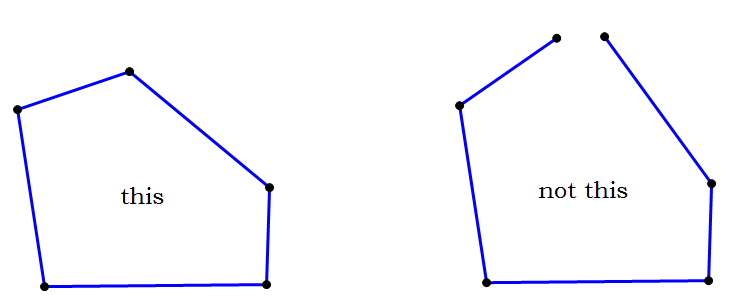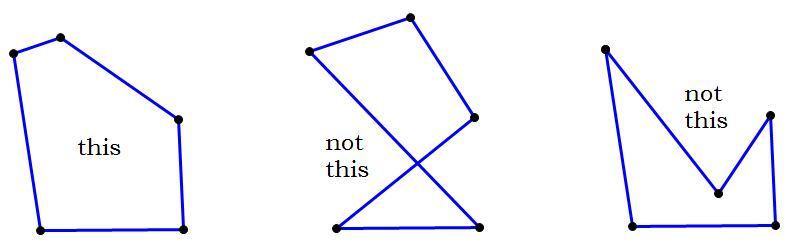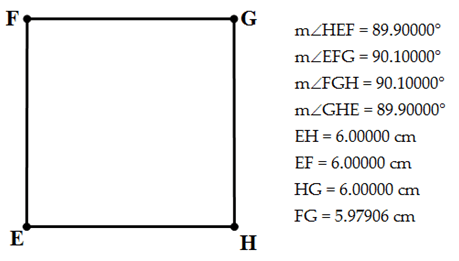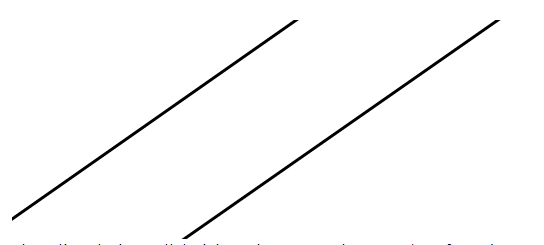Scroll

# What can I assume is true on GMAT diagrams?

Let's say you're doing quant questions for the GMAT and you encounter a diagram that looks like a square - but is it really a square? If it doesn't explicitly say it's a square in the question, can you safely assume it is or not?

Guessing at what you can or cannot assume for a diagram can take a lot of time and energy. It can also be confusing and frustrating when you're trying to solve practice problems efficiently! Don't worry, though! :D Here, you'll find information about what you can and cannot safely assume is true for diagrams on the GMAT. :)

Important note: What you can and cannot assume will depend on the type of practice problem you're doing. Diagrams in Data Sufficiency questions will have different assumptions than diagrams in Problem Solving questions on the GMAT.

Things you can comfortably assume for DS and PS questions:

1. Any line or line segment that is drawn to look straight is straight. This means there is no hidden bend or curve to the figure. It it looks straight, rest assured that it is straight! In addition, if points lie on the line (For example, a line goes through points A, B, and C) then you can say that those points are "collinear" or that they lie on the same straight line. Be careful, though: this doesn't mean a given line or segment is horizontal or vertical.

2. Any information given in the question prompt is in the diagram. If information about the figure is given in the question prompt (lengths, angles, etc), then you can assume this is reflected in the diagram.

3. If a given figure is named (For example, Figure A is a rectangle) you can assume the figure has all the properties of that type of shape. This means it’s important to know the theorems and the defined properties of shapes. You should know what it means if something is an equilateral triangle or a rhombus, so brush up on your geometry rules! In addition, if you're told that a figure is a polygon - you can assume that it is a closed shape and a convex shapeThings you can assume for DS questions only

1. The diagram is not drawn to scale. The diagram will only reflect what's written in the question prompt. It will not necessarily reflect the information that's given in the two statements. For example, if we're told that ABCD is a parallelogram in the question prompt, then the diagram will reflect this. However, if Statement 1 for the practice problem says angle ABC is 80 degrees, then it might not be accurately represented in the diagram.

Things you can assume for PS questions only

1. Most figures are drawn to scale. Diagrams on GMAT Problem Solving questions are basically drawn to scale. The only time that this isn't true is if there is a note that says "Diagram not drawn to scale" or "Diagram not necessarily to scale". If you do see a note like that then you can't really conclude a lot about how a figure looks. However, if there is no disclaimer - then you have a diagram that's drawn to scale. :) However, drawn to scale only means that it is drawn as accurately as possible. It does not mean that the diagrams are drawn 100% perfectly and accurately. Allow me to explain more below:

• Even though the diagram is drawn to scale (which is really useful overall!) - you cannot assume exact angle measurements, exact line lengths, or whether a pair of lines is parallel or perpendicular. You can only visually approximate those things.For example, the diagram above definitely looks like a square. However, none of the angles are actually 90 degrees and the sides aren't all the same length. Therefore, it's not actually a square. However, we can visually approximate and assume that these angles and lengths are similar for the purposes of a PS question - estimating this information will allow us to eliminate answer choices and verify whether an answer is in a reasonable range. In other words, these diagrams are useful when you are approximating, but not necessarily when you need a true, exact answer. :)

Things you should never assume for DS and PS questions:

1. Lines are parallel or perpendicular. Even if the lines look parallel or perpendicular, you cannot assume either on the GMAT unless it explicitly says in the question or diagram. For example, the question prompt will have the "//" symbol for parallel, the square symbol in the corner for a 90 degree angle between lines, or the "perpendicular" symbol. If they're present, then you can use all of the special geometry theorems that work for parallel or perpendicular lines. :DFor example, these two lines above look parallel. However - they aren't! They're actually 1/10 of 1 degree off of parallel - and that means that they are not. Therefore, you would not be able to use any of the special properties or theorems for parallel lines even though the lines appear to be parallel.

2. Exact measurements for lengths or angles. For example, you can't assume an angle is a right angle unless the diagram is marked with the square symbol in the corner or you're told that the diagram is a specific shape that has right angles. Similar to parallel and perpendicular lines, you can only use the geometry properties for right angles if it is actually a right angle. It will not work for an angle that is "almost a right angle" or an angle that "looks like a right angle". In addition, lengths may not be the same even if they look the same.